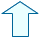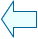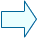GeomEtric SHAPES ON THE PLANE_1 Section: Geometry

1. THE AREA OF A SQUARE
The formula to find the area of a square is
AREA= side·side=S2

You can use the following window to help you check the formula.

 The area tells us the number of square units, i.e, the number of little squares whose sides are 1 unit long, which fit into our square ABCD.

 You can alter the length of the sides of the square by changing the side_a and side_b controls to the same value.

2. THE AREA OF A RECTANGLE
The formula to find the area of a rectangle is

AREA= side_a·side_b=a·b

 The area tells us the number of square units that there are in the rectangle ABCD, as you can see in this window.

1.- Give side a a fixed value, e.g. 3. Change the length of side b and note that the area of the rectangle form the 3 times table. Can you explain the reason for this? The Greeks used the area of a rectangle as a geometric interpretation to help them with multiplication!

 You can change the lengths of the sides of the rectangle by changing the values with the side_a and side_b control buttons. Use the Init button to restore the initial values.

3. THE AREA OF A PARALLELOGRAM
The formula to find the area of a parallelogram is

AREA= base·height=b·h

This formula is demonstrated  below. The parallelogram ABCD has the same area as a rectangle with sides b and h. As we already know how to work out the area of a rectangle we can say that the area of a parallelogram is b·h

 Use the next_step control button to watch each part of the demonstration.

1.- Now copy the demonstration with a piece of paper and a pencil.Agustín Muñoz NúñezSpanish Ministry of Education. Year 2001